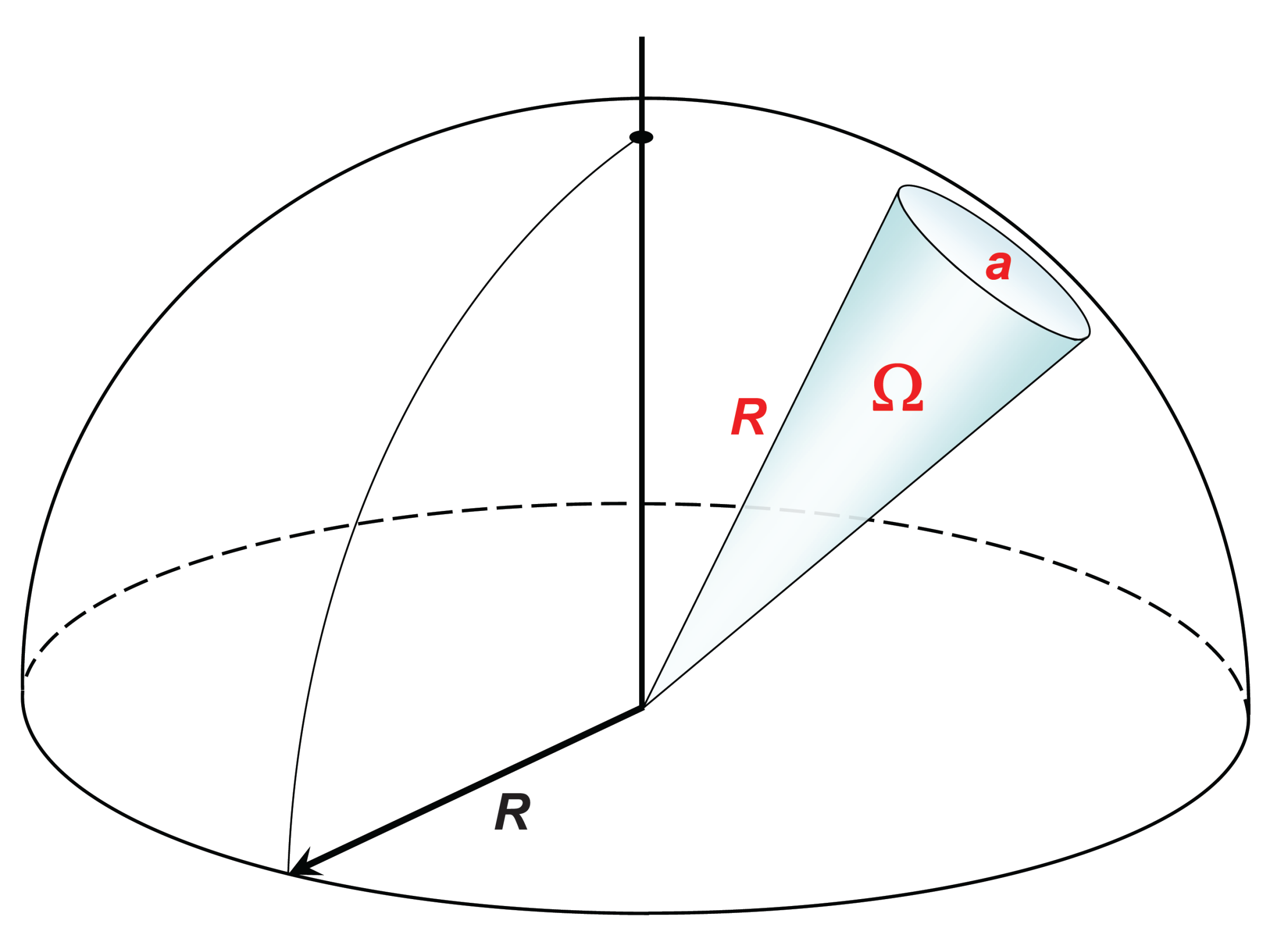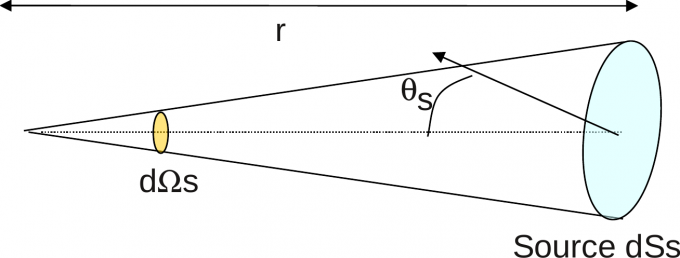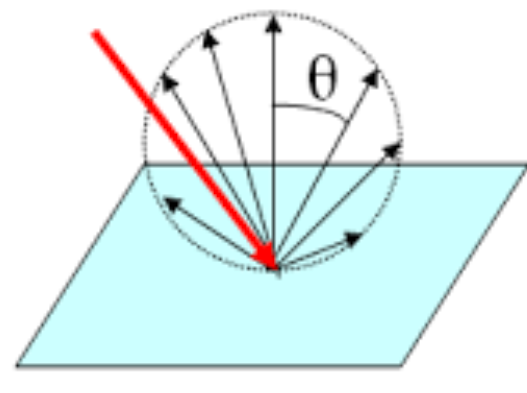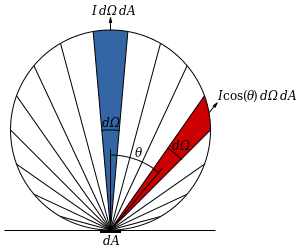# 朗伯体材质 （Lambertian） 的实现

class lambertian : public material {
public:
lambertian(const color& alb) : albedo(alb) {}

virtual optional<pair<ray, color>>
get_ray_out(const ray& r_in, const hit_rec& rec) const override {

vec3 ref_dir = rec.norm + rand_unit_vec(); // 注意这里

if(ref_dir.near_zero()) // 如果 rand_unit_vec() 等于 -rec.norm
ref_dir = rec.norm;

ray ref_ray(rec.hit_pt, ref_dir);
return make_pair(ref_ray, albedo);
}
color albedo;  // 反射率
};

## 辐射度量学

### 基本单位

$\Phi = \frac{\mathrm{d}Q}{\mathrm{d}t}$

$E = \frac{\rm{d}\Phi}{\rm{d}A}$$\Omega = \frac{a}{R^2}$

$L_\theta = \frac{\rm{d} \Phi}{\rm{d}A\cos(\theta)\rm{d}\Omega}$$I = \frac{\rm{d}\Phi}{\rm{d}\Omega}$

### 朗伯余弦定理$I_{\theta} = I_n \times \cos \theta$

## 朗伯体和漫反射

$I_{\theta} = I_n \times \cos \theta$

$L_\theta = \frac{\rm{d} \Phi}{\rm{d}A\cos(\theta)\rm{d}\Omega}$

$I_{\theta} = \frac{\rm{d}\Phi}{\rm{d}\Omega} \cos \theta$

$L_\theta = \frac{I_n \cancel{\cos \theta}}{\rm{d}A\cancel{\cos\theta}}$

## 所以，代码为什么这么写？

……
color pixel_color(0, 0, 0);
for (int s = 0; s < samples_per_pixel; ++s) {
auto u = (i + random_double()) / (image_width-1);
auto v = (j + random_double()) / (image_height-1);
ray r = cam.get_ray(u, v);
pixel_color += ray_color(r, world);
}
write_color(std::cout, pixel_color, samples_per_pixel);
……vec3 ref_dir = rec.norm + rand_unit_vec();

$I_{\theta} = I_n \times \cos \theta$